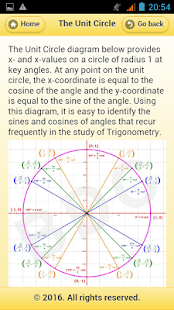The App covers topics like

1. Integrals
2. Limits
3. Geometry
4. Algebra
5. Derivatives
6. Trigonometry
7. Fundamental Theorem of Calculus
8. Integrals Definitions
9. Common Integrals
10. Properties
11. Intermediate Value Theorem
12. Relationship between the Limits
13. Limits Definitions
14. Constant Function
16. Line/Liner Function
17. Mean Value Theorem
18. Chain Rule Variants
19. Low of Tangents
20. Double Angle Formulas & many more..

Screenshots

•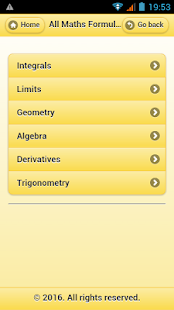•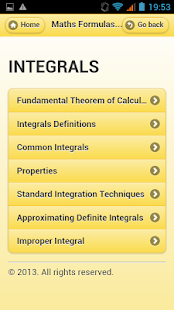•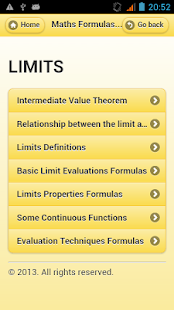•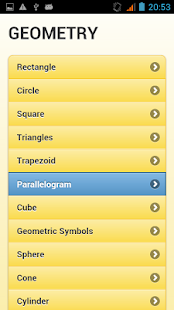•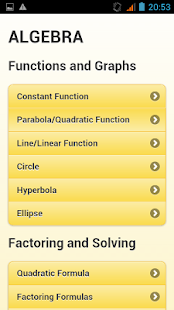•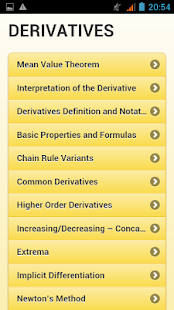•